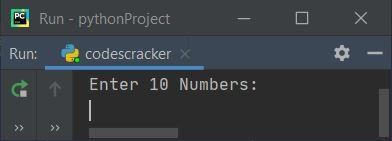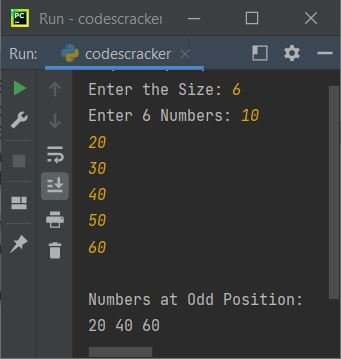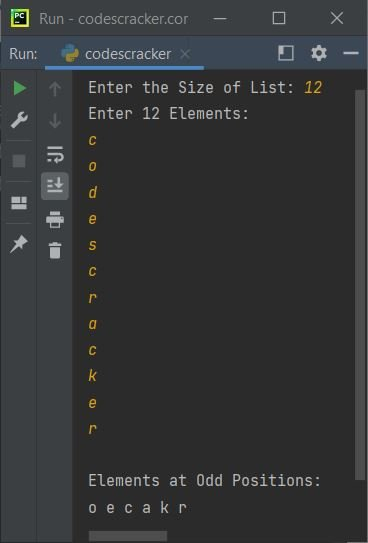# Python Program to Print Element at Odd Position in List

This article deals with some Python programs, that find and prints all elements available at odd position (index) in the list given by user at run-time. Here are the list of programs covered in this article:

• Print Elements (Numbers) at Odd Position or Index in a List of 10 Elements
• Print Numbers at Odd Position in a List of n Elements
• Print Characters at Odd Position in a List of n Elements

## Print Numbers at Odd Position in List of 10 Elements

The question is, write a Python program that find and prints all the numbers present at odd positions in a list of 10 elements. Here all 10 elements for the list must be entered by user. The program given below is answer to this question:

```arr = []
print("Enter 10 Numbers: ")
for i in range(10):
arr.insert(i, int(input()))

print("\nNumbers at Odd Positions: ")
for i in range(10):
if i%2!=0:
print(arr[i])```

Here is its sample run:Now supply the input say 1, 2, 3, 4, 5, 6, 7, 8, 9, 10 as ten numbers, to find and print all numbers that are at odd index numbers. Here index is referred as position. Let's have a look at the snapshot given below:## Print Numbers at Odd Position in List of n Elements

Basically this is the modified version of previous program. As this program allows user to define the size of list along with its elements. Then find and prints all the elements available at odd index numbers like shown in the program and its sample run given below:

```arr = list()
print(end="Enter the Size: ")
arrTot = int(input())
print(end="Enter " +str(arrTot)+ " Numbers: ")
for i in range(arrTot):
arr.append(int(input()))

print("\nNumbers at Odd Position: ")
for i in range(arrTot):
if i%2!=0:
print(end=str(arr[i]) +" ")
print()```

Here is its sample run with user input, 6 as size and 10, 20, 30, 40, 50, 60 as six numbers:## Print Characters at Odd Position in List of n Elements

This is the last program, that find and prints all characters available at odd position in a list of n characters.

```arr = []
print(end="Enter the Size of List: ")
arrSize = int(input())
print("Enter " +str(arrSize)+ " Elements: ")
for i in range(arrSize):
arr.append(input())

print("\nElements at Odd Positions: ")
for i in range(arrSize):
if i%2!=0:
print(end=str(arr[i]) + " ")
print()```

Here is its sample run with user input 12 as size of the list and c, o, d, e, s, c, r, a, c, k, e, r as twelve elements (characters) of list:Python Online Test

« Previous Program Next Program »